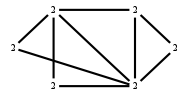# The Markov Bases Database

## G162g_bin

The binary graphical model of G162.

It is a hierarchical model of 6 variables. The dimension of the model is 19.The cardinality of the statespace is 64.

### Properties of the Markov basis

 Markov degree 2 248

 degree 2 248

The model has the following properties:

• All variables are binary.
• It is a graphical model.
• The semigroup is normal.### FILES

 Markov basis: sufficient statistics matrix: G162g_bin.mar (46.75 kb) G162g_bin.mat (4.04 kb) G162g_bin.mod (49 b) G162g_bin.tar.gz (7.36 kb)